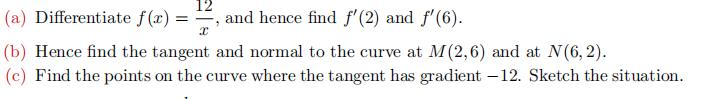### Still have math questions?

Algebra
Question(a) Differentiate $$f ( x ) = \frac { 12 } { x }$$ , and hence find $$f ^ { \prime } ( 2 )$$ and $$f ^ { \prime } ( 6 )$$

(b) Hence find the tangent and normal to the curve at $$M ( 2,6 )$$ and at $$N ( 6,2 )$$ .

(c) Find the points on the curve where the tangent has gradient $$- 12$$ . Sketch the situation.

$$\left. \begin{array} { l } { \text { 8(a) } f ^ { \prime } ( x ) = - \frac { 12 } { x ^ { 2 } } , f ^ { \prime } ( 2 ) = - 3 , f ^ { \prime } ( 6 ) = - \frac { 1 } { 3 } } \\ { \left. \begin{array} { l } { \text { (b) At } M ( 2,6 ) , \text { tangent: } y = - 3 x + 12 , \text { normal: } } \\ { x - 3 y + 16 = 0 . \text { At } N ( 6,2 ) , \text { tangent: } y = - \frac { 1 } { 3 } x + 4 } \\ { \text { normal: } y = 3 x - 16 . \quad \text { (c) } ( 1,12 ) \text { and } ( - 1 , - 12 ) } \end{array} \right. } \end{array} \right.$$